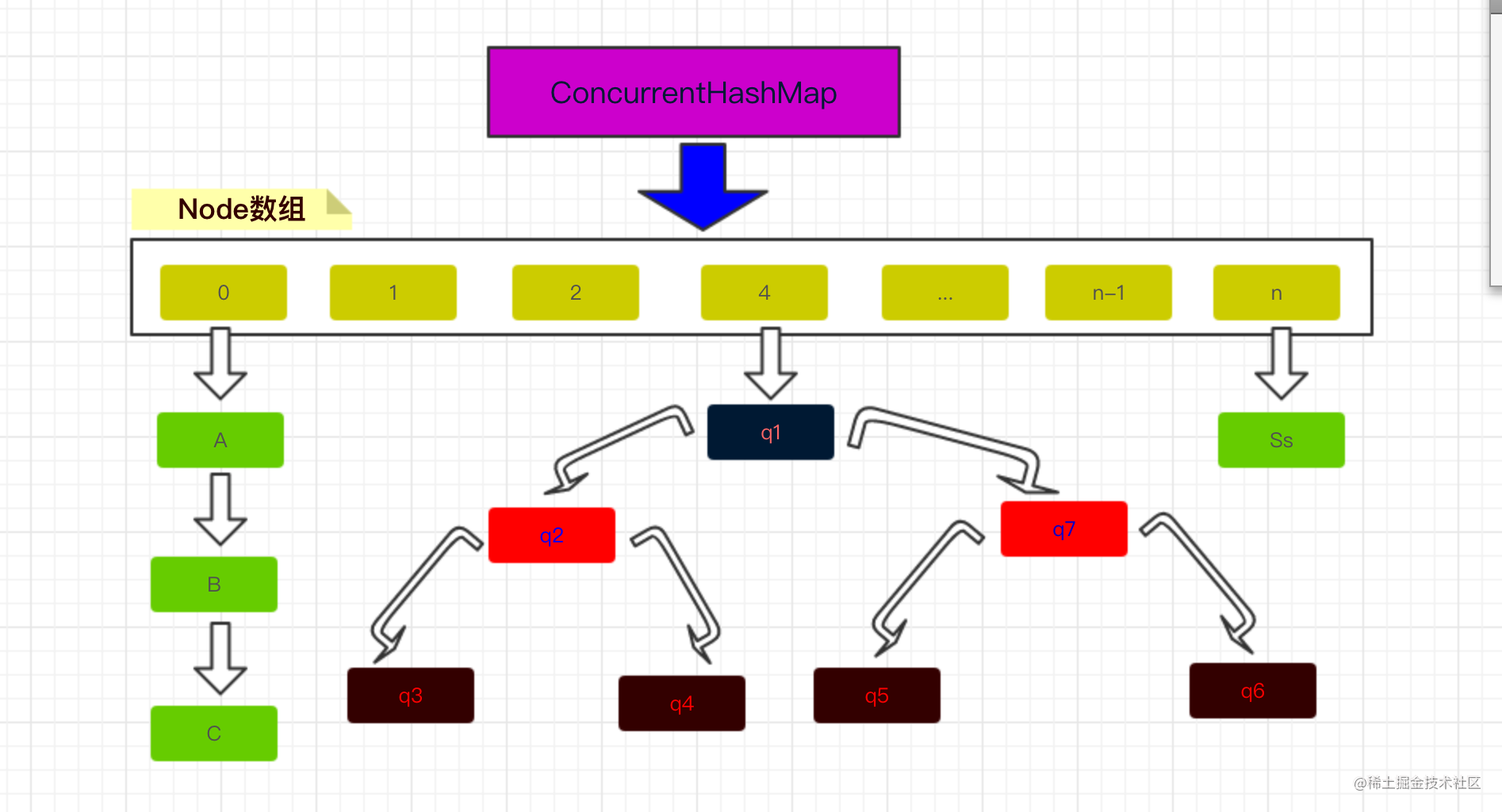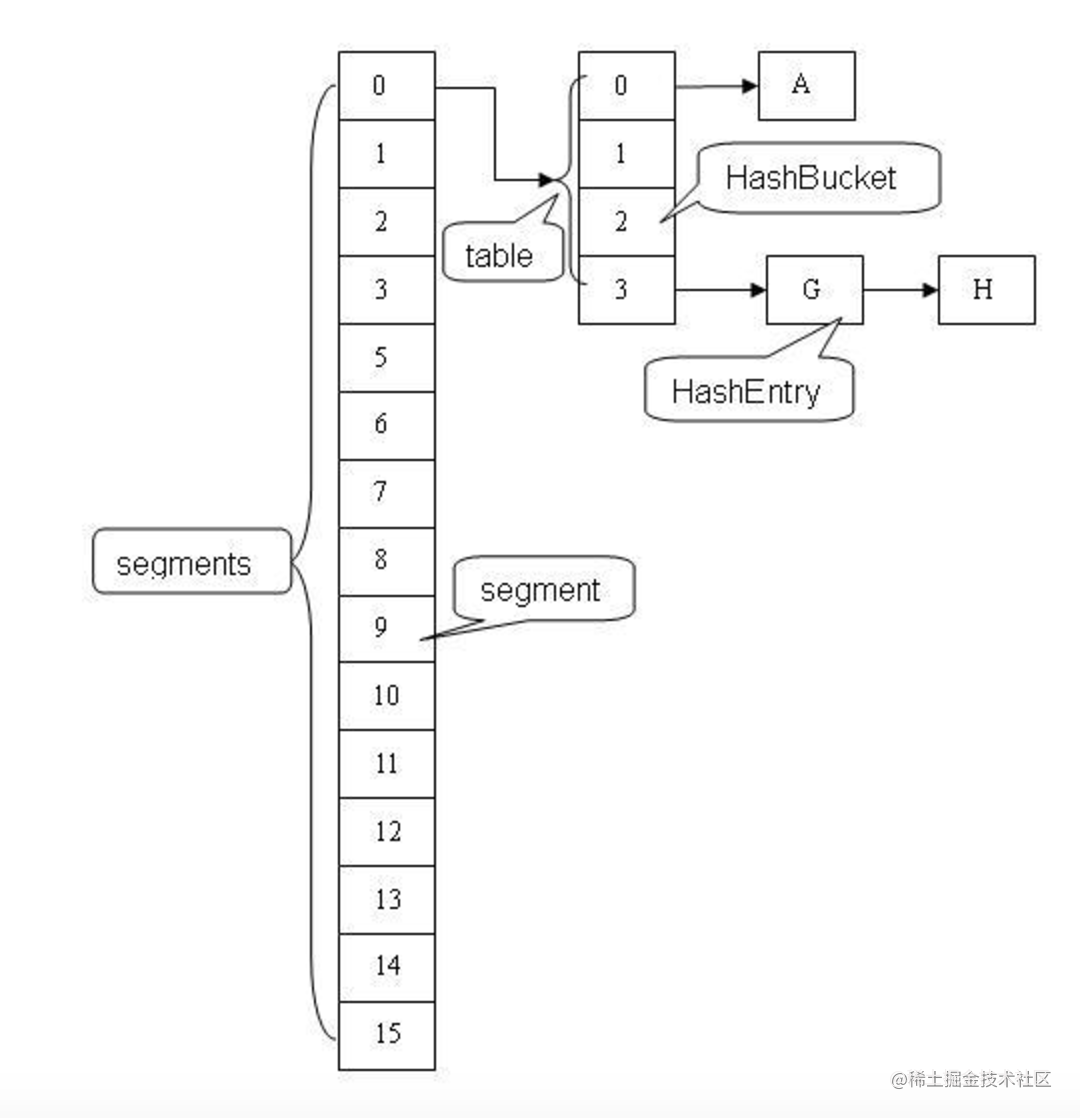# Java 8 ConcurrentHashMap源码分析

## 导入

ConcurrentHashMap是HashMap的线程安全版本的实现版本，关于HashMap的分析总结，可以参考文章Java 8 HashMap源码分析。本文将基于java 8中的Java 8 实现来分析ConcurrentHashMap，与之前版本的ConcurrentHashMap实现来看，java 8中做了较大调整，本文仅分析java 8的实现，java 8 之前的实现暂不做分析。为了更好的导入本文，首先展示一下ConcurrentHashMap的结构，请看下面的图片：ConcurrentHashMap结构图

## ConcurrentHashMap详解

### 哈希桶Table初始化

``````
private final Node<K,V>[] initTable() {
Node<K,V>[] tab; int sc;
while ((tab = table) == null || tab.length == 0) {
if ((sc = sizeCtl) < 0)
Thread.yield(); // lost initialization race; just spin
else if (U.compareAndSwapInt(this, SIZECTL, sc, -1)) {
try {
if ((tab = table) == null || tab.length == 0) {
int n = (sc > 0) ? sc : DEFAULT_CAPACITY;
@SuppressWarnings("unchecked")
Node<K,V>[] nt = (Node<K,V>[])new Node<?,?>[n];
table = tab = nt;
sc = n - (n >>> 2);
}
} finally {
sizeCtl = sc;
}
break;
}
}
return tab;
}

``````

``````
/**
* Table initialization and resizing control.  When negative, the
* table is being initialized or resized: -1 for initialization,
* else -(1 + the number of active resizing threads).  Otherwise,
* when table is null, holds the initial table size to use upon
* creation, or 0 for default. After initialization, holds the
* next element count value upon which to resize the table.
*/
private transient volatile int sizeCtl;

``````

sizeCtl是一个用于同步多个线程的共享变量，如果它的当前值为负数，则说明table正在被某个线程初始化或者扩容，所以，如果某个线程想要初始化table或者对table扩容，需要去竞争sizeCtl这个共享变量，获得变量的线程才有许可去进行接下来的操作，没能获得的线程将会一直自旋来尝试获得这个共享变量，所以获得sizeCtl这个变量的线程在完成工作之后需要设置回来，使得其他的线程可以走出自旋进行接下来的操作。而在initTable方法中我们可以看到，当线程发现sizeCtl小于0的时候，他就会让出CPU时间，而稍后再进行尝试，当发现sizeCtl不再小于0的时候，就会通过调用方法compareAndSwapInt来讲sizeCtl共享变量变为-1，以告诉其他试图获得sizeCtl变量的线程，目前正在由本线程在享用该变量，在我完成我的任务之前你可以先休息一会，等会再来试试吧，我完成工作之后会释放掉的。而其他的线程在发现sizeCtl小于0的时候就会理解这种交流，他们会让出cpu时间，等待下次调度再来尝试获取sizeCtl来进行自己的工作。在完成初始化table的任务之后，线程需要将sizeCtl设置成可以使得其他线程获得变量的状态，这其中还有一个地方需要注意，就是在某个线程通过U.compareAndSwapInt方法设置了sizeCtl之前和之后进行了两次check，来检测table是否被初始化过了，这种检测是必须的，因为在并发环境下，可能前一个线程正在初始化table但是还没有成功初始化，也就是table依然还为null，而有一个线程发现table为null他就会进行竞争sizeCtl以进行table初始化，但是当前线程在完成初始化之后，那个试图初始化table的线程获得了sizeCtl，但是此时table已经被初始化了，所以，如果没有再次判断的话，可能会将之后进行put操作的线程的更新覆盖掉，这是极为不安全的行为。

### ConcurrentHashMap查询记录方法详解

``````
public V get(Object key) {
Node<K,V>[] tab; Node<K,V> e, p; int n, eh; K ek;
if ((tab = table) != null && (n = tab.length) > 0 &&
(e = tabAt(tab, (n - 1) & h)) != null) {
if ((eh = e.hash) == h) {
if ((ek = e.key) == key || (ek != null && key.equals(ek)))
return e.val;
}
else if (eh < 0)
return (p = e.find(h, key)) != null ? p.val : null;
while ((e = e.next) != null) {
if (e.hash == h &&
((ek = e.key) == key || (ek != null && key.equals(ek))))
return e.val;
}
}
return null;
}

``````

``````
static final int TREEBIN   = -2; // hash for roots of trees

TreeBin(TreeNode<K,V> b) {
super(TREEBIN, null, null, null);

......
}

``````

``````
static class Node<K,V> implements Map.Entry<K,V> {
final int hash;
final K key;
volatile V val;
volatile Node<K,V> next;

....
}

/**
* The array of bins. Lazily initialized upon first insertion.
* Size is always a power of two. Accessed directly by iterators.
*/
transient volatile Node<K,V>[] table;

``````

### ConcurrentHashMap插入记录方法详解

``````
public V put(K key, V value) {
return putVal(key, value, false);
}

/** Implementation for put and putIfAbsent */
final V putVal(K key, V value, boolean onlyIfAbsent) {
if (key == null || value == null) throw new NullPointerException();
int binCount = 0;
for (Node<K,V>[] tab = table;;) {
Node<K,V> f; int n, i, fh;
if (tab == null || (n = tab.length) == 0)
tab = initTable();
else if ((f = tabAt(tab, i = (n - 1) & hash)) == null) {
if (casTabAt(tab, i, null,
new Node<K,V>(hash, key, value, null)))
break;                   // no lock when adding to empty bin
}
else if ((fh = f.hash) == MOVED)
tab = helpTransfer(tab, f);
else {
V oldVal = null;
synchronized (f) {
if (tabAt(tab, i) == f) {
if (fh >= 0) {
binCount = 1;
for (Node<K,V> e = f;; ++binCount) {
K ek;
if (e.hash == hash &&
((ek = e.key) == key ||
(ek != null && key.equals(ek)))) {
oldVal = e.val;
if (!onlyIfAbsent)
e.val = value;
break;
}
Node<K,V> pred = e;
if ((e = e.next) == null) {
pred.next = new Node<K,V>(hash, key,
value, null);
break;
}
}
}
else if (f instanceof TreeBin) {
Node<K,V> p;
binCount = 2;
if ((p = ((TreeBin<K,V>)f).putTreeVal(hash, key,
value)) != null) {
oldVal = p.val;
if (!onlyIfAbsent)
p.val = value;
}
}
}
}
if (binCount != 0) {
if (binCount >= TREEIFY_THRESHOLD)
treeifyBin(tab, i);
if (oldVal != null)
return oldVal;
break;
}
}
}
return null;
}

``````

### ConcurrentHashMap记录数量更新

``````
private final void addCount(long x, int check) {
CounterCell[] as; long b, s;
if ((as = counterCells) != null ||
!U.compareAndSwapLong(this, BASECOUNT, b = baseCount, s = b + x)) {
CounterCell a; long v; int m;
boolean uncontended = true;
if (as == null || (m = as.length - 1) < 0 ||
(a = as[ThreadLocalRandom.getProbe() & m]) == null ||
!(uncontended =
U.compareAndSwapLong(a, CELLVALUE, v = a.value, v + x))) {
return;
}
if (check <= 1)
return;
s = sumCount();
}
if (check >= 0) {
Node<K,V>[] tab, nt; int n, sc;
while (s >= (long)(sc = sizeCtl) && (tab = table) != null &&
(n = tab.length) < MAXIMUM_CAPACITY) {
int rs = resizeStamp(n);
if (sc < 0) {
if ((sc >>> RESIZE_STAMP_SHIFT) != rs || sc == rs + 1 ||
sc == rs + MAX_RESIZERS || (nt = nextTable) == null ||
transferIndex <= 0)
break;
if (U.compareAndSwapInt(this, SIZECTL, sc, sc + 1))
transfer(tab, nt);
}
else if (U.compareAndSwapInt(this, SIZECTL, sc,
(rs << RESIZE_STAMP_SHIFT) + 2))
transfer(tab, null);
s = sumCount();
}
}
}

``````

### ConcurrentHashMap移除记录操作

``````
public V remove(Object key) {
return replaceNode(key, null, null);
}

final V replaceNode(Object key, V value, Object cv) {
for (Node<K,V>[] tab = table;;) {
Node<K,V> f; int n, i, fh;
if (tab == null || (n = tab.length) == 0 ||
(f = tabAt(tab, i = (n - 1) & hash)) == null)
break;
else if ((fh = f.hash) == MOVED)
tab = helpTransfer(tab, f);
else {
V oldVal = null;
boolean validated = false;
synchronized (f) {
if (tabAt(tab, i) == f) {
if (fh >= 0) {
validated = true;
for (Node<K,V> e = f, pred = null;;) {
K ek;
if (e.hash == hash &&
((ek = e.key) == key ||
(ek != null && key.equals(ek)))) {
V ev = e.val;
if (cv == null || cv == ev ||
(ev != null && cv.equals(ev))) {
oldVal = ev;
if (value != null)
e.val = value;
else if (pred != null)
pred.next = e.next;
else
setTabAt(tab, i, e.next);
}
break;
}
pred = e;
if ((e = e.next) == null)
break;
}
}
else if (f instanceof TreeBin) {
validated = true;
TreeBin<K,V> t = (TreeBin<K,V>)f;
TreeNode<K,V> r, p;
if ((r = t.root) != null &&
(p = r.findTreeNode(hash, key, null)) != null) {
V pv = p.val;
if (cv == null || cv == pv ||
(pv != null && cv.equals(pv))) {
oldVal = pv;
if (value != null)
p.val = value;
else if (t.removeTreeNode(p))
setTabAt(tab, i, untreeify(t.first));
}
}
}
}
}
if (validated) {
if (oldVal != null) {
if (value == null)
return oldVal;
}
break;
}
}
}
return null;
}

``````

### ConcurrentHashMap的size方法详解

``````
public int size() {
long n = sumCount();
return ((n < 0L) ? 0 :
(n > (long)Integer.MAX_VALUE) ? Integer.MAX_VALUE :
(int)n);
}

final long sumCount() {
CounterCell[] as = counterCells; CounterCell a;
long sum = baseCount;
if (as != null) {
for (int i = 0; i < as.length; ++i) {
if ((a = as[i]) != null)
sum += a.value;
}
}
return sum;
}

``````

ConcurrentHashMap的记录数量需要结合baseCount和counterCells数组来得到，通过累计两者的数量即可获得当前ConcurrentHashMap中的记录总量。

## java7中的ConcurrentHashMap实现java7中的ConcurrentHashMap结构图